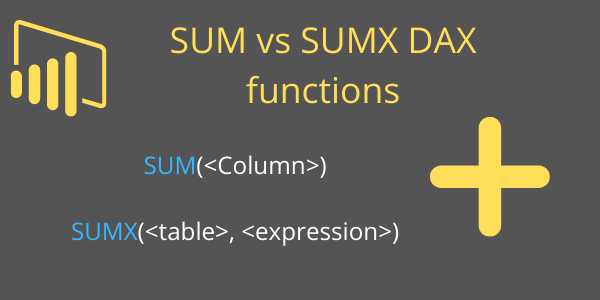Home » Interviews Q & A » SUM Vs SUMX : DAX Difference

# SUM Vs SUMX : DAX DifferenceIn Power BI, both SUM and SUMX are aggregation functions used to calculate the sum of values.

### SUM DAX Function

The SUM function is a basic aggregate function that calculates the sum of a set of values. It takes a column of values as an argument and returns the sum of those values.

```Syntax: SUM(<Column>)
Example: Total_Sales = SUM(Orders[Sales])```

This will return the sum of all the values in the `Sales` column of the `Orders` table.

### SUMX DAX Function

The SUMX function is an iterative calculation function that calculates a sum of values for each value in a table. It takes two arguments: an expression that calculates a value for each row in a table, and the name of the table.

```Syntax- SUMX(<table>, <expression>)
Example-  Total_Sale = SUMX(Orders, Orders[Sales] * Order[Quantity] )```

The SUMX function calculates the expression for each row in the table and returns the sum of those values.

In short, the SUM function is used for simple sum calculations, while the SUMX function is used for more complex calculations that require iteration over a table.

If you want to understand in details refer this post- SUM Vs SUMX DAX